# Factored Form to Graph

To graph a quadratic function, it is helpful to know the overall shape (parabola) and a few key points.

The points that are most useful are:

•-intercept
• vertex
•-intercepts (if there are any)
• symmetry of the-intercept

These can all be calculated directly from the factored form.

## ExampleLet’s begin by drawingandaxes, and labelling theaxis fromto. We leave theaxis blank for now until we know how far down or up the vertex is.Theintercept occurs when thecoordinate is zero. Therefore when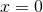:The-intercept is the point.

The-intercepts occur when thecoordinate is zero. To locate them, we need to solve the equationThis turns out to be quite straightforward. When any two numbers multiply to give zero, one or the other or both of the numbers must be zero. Therefore, if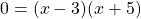then eitheror.

The-intercepts occur at the pointsand.

Thecoordinate of the vertex is right in the middle of the intercepts. To find the middle of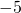and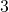, we can do a variety of calculations. One way is to add them together and divide by 2 (find the average of the two numbers).Now to find thecoordinate of the vertex, we simply substitute thecoordinate into the equation for. When: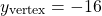The coordinates of the vertex are.

We now know that the lowest point on the parabola is at, and so let’s use a scale of 5 on theaxis to accommodate the vertex.

Let’s plot all these points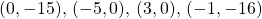on the graph.The last point to plot is the symmetry of theintercept. If you imagine a mirror line through the vertex, and reflect theintercept over that to plot a fifth point.We are now ready to draw the smoothest parabola curve through the plotted points, making sure to round off the vertex and not make it too pointy.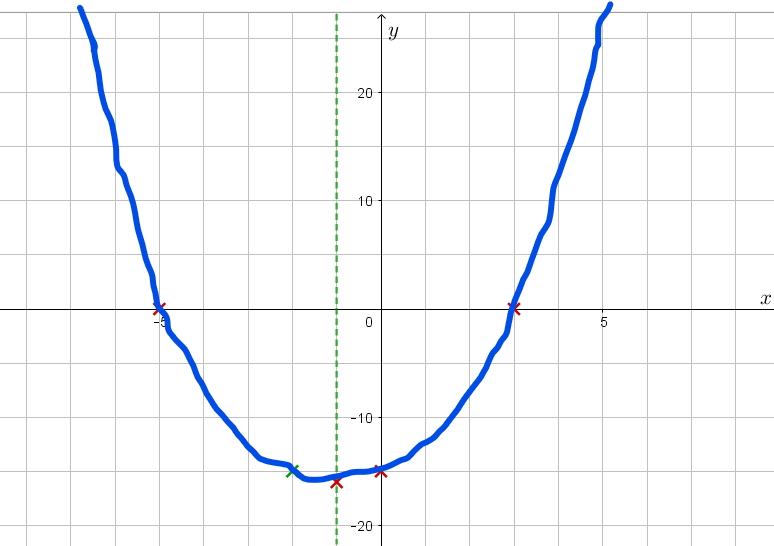Note that a function such asorwill generate a parabola that turns downwards rather than upwards.

Note that if it is not possible to factor a quadratic expression, we must use a different technique for graphing. If there are-intercepts we can find them using the quadratic formula. If there are no-intercepts, then we are left with just the vertex, the-intercept and the symmetry of the-intercept. Since we can’t ‘add the roots then divide by two’ to get the-coordinate of the vertex, we need a different technique. Several techniques to find the vertex are discussed on the page Find the Vertex, which the last page of this unit.